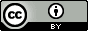## Saturday, April 16, 2016

### Computing pKa values for molecules with several ionizable groups

We're working on pKa prediction using semiempirical methods and need to compute pKa values  for molecules with several ionizable groups. Here are my current thoughts so far.

Background: one ionizable group
If there is only one tritrateable site
$$\mathrm{BH \rightleftharpoons B + H^+} \ \ \ K=\mathrm{\frac{[B][H^+]}{[BH]}}$$
then the fraction of $\mathrm{BH}$ molecules $f_{\mathrm{BH}}$ is $$\begin{split} f_{\mathrm{BH}} & =\mathrm{\frac{[BH]}{[B]+[BH]} } \\ & = \mathrm{\frac{[B]}{[B]}\frac{[BH]/[B]}{1+[BH]/[B]} } \\ & = \mathrm{\frac{[H^+]/K}{1+[H^+]/K} } \\ & = \mathrm{\frac{10^{p\textit{K}-pH}}{1+10^{p\textit{K}-pH}} } \end{split}$$ where $$\mathrm{pH = -log[H^+] \implies [H^+] = 10^{-pH}}$$
and similarly for $K$.

From this we can see that the pK value is the pH value for which $f_{\mathrm{BH}}$ = 1/2. So you compute the pK value from the standard free energy difference
$$\text{p}K =\left( \Delta G^\circ(\mathrm{B})+\Delta G^\circ(\mathrm{H^+})- \Delta G^\circ(\mathrm{BH})\right)/RT\ln(10)$$
and you're done.

Two ionizable groups
For a molecule with two titrateable groups ($\mathrm{HB_\alpha B_\beta H}$) and the following equilibrium constants $$\mathrm{HBBH \rightleftharpoons BBH + H^+} \ \ K_{\alpha1}$$ $$\mathrm{HBBH \rightleftharpoons HBB + H^+} \ \ K_{\beta1}$$ $$\mathrm{HBB \rightleftharpoons BB + H^+} \ \ K_{\alpha0}$$ $$\mathrm{BBH \rightleftharpoons BB + H^+} \ \ K_{\beta0}$$ The probability of, for example, $\mathrm{BBH}$ is $$f_{\mathrm{BBH}} =\mathrm{\frac{[BBH]}{[BB]+[BBH]+[HBB]+[HBBH]}= \frac{[BBH]}{\textit{P}}}$$ $f_{\mathrm{BBH}}$ can be rewritten in terms of pK values $$f_{\mathrm{BBH}} = \mathrm{\frac{[BBH]/[BB]}{\textit{P}/[BB]} = \frac{10^{p\textit{K}_{\beta0}-pH}}{\textit{P}/[BB]}}$$ where $$\mathrm{ \textit{P}/[BB] = 1+10^{p\textit{K}_{\alpha0}-pH}+10^{p\textit{K}_{\beta0}-pH}+ 10^{p\textit{K}_{\alpha0}+p\textit{K}_{\beta1}-2pH}}$$ Similarly, $$f_{\mathrm{HBB}} = \mathrm{\frac{10^{p\textit{K}_{\alpha0}-pH}}{\textit{P}/[BB]}}$$ and $$f_{\mathrm{HBBH}} = \mathrm{\frac{10^{p\textit{K}_{\alpha0}+p\textit{K}_{\beta1}-2pH}}{\textit{P}/[BB]}}$$  The apparent pK value of the $\alpha$ group ($\mathrm{p}K_{\alpha}$) is the pH at which its protonation probability $$f_\alpha =f_{\mathrm{HBB}} + f_{\mathrm{HBBH}}$$ is 1/2 and similarly for the $\beta$ group.  So compute the microscopic pK values (Eq 4-7), then $f_\alpha$ and $f_\beta$ as a function of pH, and then $\mathrm{p}K_{\alpha}$ and $\mathrm{p}K_{\beta}$

If one of the groups (say $\alpha$) titrates at a significantly lower pH than the other ($\mathrm{p}K_{\alpha1} << \mathrm{p}K_{\beta1}$) then $\mathrm{p}K_{\alpha}=\mathrm{p}K_{\alpha1}$ and $\mathrm{p}K_{\beta}=\mathrm{p}K_{\beta0}$ and it is not necessary to compute the free energy of $\mathrm{HBB}$, but it can be hard to determine this in advance.  Similarly, if there is no significant interaction between the sites then $\mathrm{p}K_{\alpha}=\mathrm{p}K_{\alpha1}=\mathrm{p}K_{\alpha0}$ and $\mathrm{p}K_{\beta}=\mathrm{p}K_{\beta1}=\mathrm{p}K_{\beta0}$ and one can skip one of the protonation states.

For $N$ ionizable groups one has to determine $2^N$ microscopic pKa values, which quickly gets out of hand if one has to do a conformational search for each protonation state and the molecule is large.

Related post
Generating protonation states and conformations## Wednesday, April 13, 2016

### Reviewing for PeerJ: it's the little (and the not so little) things

I just did my first review for PeerJ and it was a real pleasure because there are a lot of "little things" that make your reviewing life easier:

1. Figures/tables are in the text and, get this, the captions are immediately above/below the corresponding figure/table.  Some other journals also do this, but not enough.

2. I annotate the mss in a pdf reader and usually this is a frustrating experience since the publisher generated pdf has all sorts of "quirks" that make highlighting and copying text hit and miss.  The previous pdf I reviewed turned every page with a figure into an image!  Annotating/copying in the PeerJ pdf worked flawlessly.

3. The pdf contained a 3 front pages with the due date, a summary of the review criteria, a link to the page with the supplementary material, and a link to the page where I should submit my review.  No hunting around for the email with the link! I teared up a little bit when I saw that.

Other "little things" include stuff like not having to rank the perceived importance or impact of the work on some bogus 1-10 scale, a strict policy on making the raw data available, and a button to click to make my review non-anonymous.## Thursday, April 7, 2016

### Why I use Twitter: one scientist's perspective

Tomorrow I am giving a short talk on why and how I use Twitter to public relations people at the University.  I have 20 min + 10  min questions, but am aiming for a 10 min talk + 20 min questions.## Tuesday, April 5, 2016

### ACS Omega was too expensive

2018.01.23 update: I just noticed that the APC has been lowered to \$750 + 1 year ACS membership for corresponding author. This is actually a reasonably good deal, so I am changing title. Disclaimer: I applied to be a co-editor of this journal and was not selected. ACS Omega has just announced its APCs: \$1500 (\$2000) under the ACS Authors Choice (CC-BY or CC-BY-NC-ND) license for members and an additional \$500 for non-members (since full membership costs $162, this is the real additional cost for one yearly publication). The ACS Authors Choice license is not open access: under the ACS Authors Choice license you assign copyright to the ACS. While For non-commercial research and education purposes only, users may access, download, copy, display and redistribute articles as well as adapt, translate, text and data mine content contained in articles, ... you still can't, for example, use a figure from such an article in a book chapter without the ACS permission. Also, the ACS can, for example, sell your article or your figures. So the cost for to publish OA is ACS Omega is$2000.  That's more expensive than other impact neutral OA journals: PLoS One (\$1495), Scientific Reports (\$1495), F1000Research (\$1000), Rio Journal (\$850), PeerJ (\$100/author, Bio-only), and Royal Society Open Science ($0, for now).

Since all journals are impact neutral and provide quick review (AFAIK) price is the main considerations and I see no reason to pay more for to publish in ACS Omega.